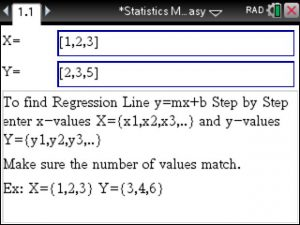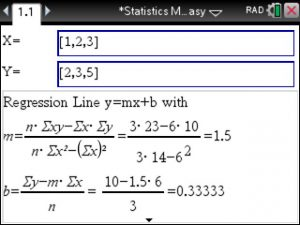## Lower & Upper Inner (Outer) Fence , IQR , 5 Number Summary (Tinspire CX)

If your Stats teacher is asking you to compute things such as:

• Lower & Upper Inner (Outer) Fence
• IQR
• 5 Number Summary

and you have a Tinspire CX CAS then head over to Statistics Made Easy which actually makes Statistics a lot easier: Finding Mean, Median, Mode, Standard Deviation, Variance, Fences, IQR, Statistical Tests, Distributions, Confidence Intervals and a whole lot more …

## Correlation Coefficient and Coefficient of Determination using the TiNSpire – Step by Step

Linear Regression you just type in the given x and y values. Next the linear regression is computed for you along with the  Correlation Coefficient and the Coefficient of Determination.

That simple.Posted on Categories statistics, tinspirecx

## ▷Everything works like a charm. Just purchased the statistics app for the TiNSpire CX

Hey Mike!

All is well!

You helped me over text a week ago!

Everything works like a charm.

Just purchased the statistics app and just activated

That works as well.

Posted on Categories customer feedback

## Step by Step Statistics app for the Ti-Nspire CX available nowWatch this Youtube Video for an overview and examples at https://youtu.be/1HKbdf4zvvM

Shows Step by Step solutions for most Statistics and Probability topics.

• Step by Step Confidence Intervals for mean, proportion, 2 means, 2 proportions, variance, slope, etc.
• Compute Sample Sizes for Confidence Intervals.
• Step by Step Tests of Significance (Z-Tests, T-Tests, ChiSquare Goodness of Fit and Independence tests, 2-sample T tests, 2 proportion Z tests, for Variance, etc)
• Step by Step Regression.
• Read about Significant Tests: Type 1 Error, Type 2 Error, Power of a Test.
• Much easier handling than other Statistics packages: Choose to enter Data or Statistic values. Select Significant/Confidence Levels easily.
• Distributions: compute probabilities, etc.
• Includes Binomial, Geometric, Normal, Student-t, Exponential, Poisson, Multinomial, Hypergeometric, F-, Chi-Square and more Distributions.
• Probability Checker for independent events, disjoint events. Also solves P(A or B)=P(A)+P(B)-P(A and B) for the unknown.
• Regressions: Linear , Quadratic, Cubic, Quartic, Sinusoidal, Logarithmic, Exponential, Power, MedMed
• Step by Step Derive the Coefficients for a Linear Regression Line, the Coefficient of Determination and the Correlation Coefficient.
• Also: Z-score Solver, Random Number Generator, etc
• 1 or 2 variable stats on mean, mode, median, standard deviation, variance, range
Posted on Categories statistics

## Step by Step Statistics App now available for the TI-Nspire CX CAS

Hi TI-Nspire users: Step by Step Statistics problem solving involving

-Statistical Analysis

-Mean, Mode, Median, Std Deviation, Variance

-Linear , Quadratic and 6 other Regressions

-Confidence Intervals

-Statistical Tests

-Normal Distribution, Binomial Distribution and 6 other Distributions

-Conditional Probability

and much more..

Free trials.